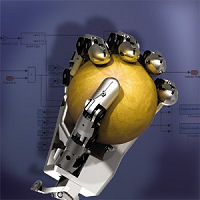Search IntMath
Close

450+ Math Lessons written by Math Professors and Teachers

5 Million+ Students Helped Each Year

1200+ Articles Written by Math Educators and Enthusiasts

Simplifying and Teaching Math for Over 23 Years

# Math software improves prosthetic devices

By Murray Bourne, 08 Dec 2009

Here's an interesting real-life application of math.

Prosthetic devices help people if they have been injured in an accident and have lost an arm or leg.In the article Developing the World’s Most Advanced Prosthetic Arm Using Model-Based Design, we read how Matlab mathematics software is being used to improve the movement of prosthetic devices.

The user is able to control the device using inputs from his brain and gets sensory feedback so he knows what he is doing. In the past, users would easily crush objects they were trying to hold, because there was not enough feedback.

Now, there's a worthwhile application of math.

Be the first to comment below.

### Comment Preview

HTML: You can use simple tags like <b>, <a href="...">, etc.

To enter math, you can can either:

1. Use simple calculator-like input in the following format (surround your math in backticks, or qq on tablet or phone):
a^2 = sqrt(b^2 + c^2)
(See more on ASCIIMath syntax); or
2. Use simple LaTeX in the following format. Surround your math with $$ and $$.
$$\int g dx = \sqrt{\frac{a}{b}}$$
(This is standard simple LaTeX.)

NOTE: You can mix both types of math entry in your comment.